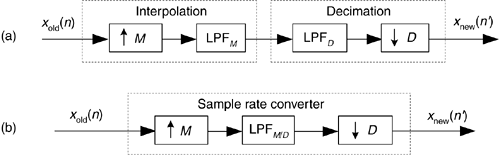# COMBINING DECIMATION AND INTERPOLATION

Although changing sampling rates, through decimation or interpolation, by integer factors can be useful, what can we do if we need a sample rate change that is not an integer? The good news is we can implement sample rate conversion by any rational fraction M/D with interpolation by an integer factor of M followed by decimation by an integer factor of D. Because the ratio M/D can be obtained as accurately as we want, with the correct choice of integers M and D, we can change sample rates by almost any factor in practice. For example, a sample rate increase by a factor of 7.125 can be performed by an interpolation of M = 57 followed by a decimation of D = 8, because 7.125 = 57/8.

This M/D sample rate change is illustrated as the processes shown in Figure 10-7(a). The upsampling operationM means insert M – 1 zero-valued samples between each xold(n) sample. The neat part here is that the computational burden of changing the sample rate by the ratio of M/D is less than the sum of an individual interpolation followed by an individual decimation. That's because we can combine the interpolation filter LPFM and the decimation filter LPFD into a single filter, shown as LPFM/D in Figure 10-7(b). The process in Figure 10-7(b) is normally called a sample rate converter because if M > D, we have interpolation, and when D > M, we have decimation. (The filter LPFM/D is often called a multirate filter.) Filter LPFM/D must sufficiently attenuate the interpolation spectral images so they don't contaminate our desired signal beyond acceptable limits after decimation.

Figure 10-7. Sample rate conversion by a rational factor: (a) combination of interpolation and decimation; (b) sample rate conversion with a single lowpass filter.Notice that the frequency response of LPFM/D must be designed so the beginning of its stopband frequency is less than fnew/2 in order to avoid aliasing after the decimation. The stopband attenuation of LPFM/D must be great enough so the attenuated images do not induce intolerable levels of noise when they're aliased by decimation into the final band of 0 to fnew/2 Hz.

Again, our interpolator/decimator problem is an exercise in lowpass filter design. All the knowledge and tools we have to design lowpass filters can be applied to this task. In software interpolator/decimator design, we want our lowpass filter algorithm to prevent aliasing images and be fast in execution time. For hardware interpolator/decimators, we strive to implement designs optimizing the conflicting goals of high performance (minimum aliasing), simple architecture, high data throughput speed, and low power.

The filtering in sample rate conversion, as we've presented it, is sadly inefficient. Think about interpolating a signal sequence by a factor of 4/3; we'd stuff three zero-valued samples into the original time sequence and apply it to a lowpass filter. Three fourths of the filter multiplication products would be zero. Next we'd discard two thirds of our filter output values. Very inefficient! Fortunately, there are special sample rate conversion filters, called digital polyphase filters, that avoid these inefficiencies. So, let's introduce polyphase filters, and then discuss a special filter, known as a cascaded integrator-comb filter, that's beneficial in hardware sample rate conversion applications.AmazonPrev don't be afraid of buying books Next